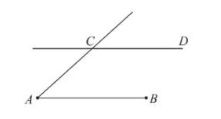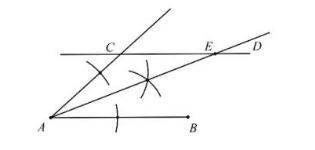(1) 作 $\angle C A B$ 的角平分线 $A E$ 交直线 $C D$ 于点 $E$ ( 尺规作图, 保留作图痕迹, 不写作法);
(2) 在 (1) 的条件下, 若 $A E$ 的中点为 $F$, 连接 $B F$ 并延长交直线 $C D$ 于点 $G$, 请用等式表 示线段 $A B, A C, C G$ 之间的数量关系:(1)(2) $AB=AC+CG$
①点击 首页查看更多试卷和试题 , 点击查看 本题所在试卷